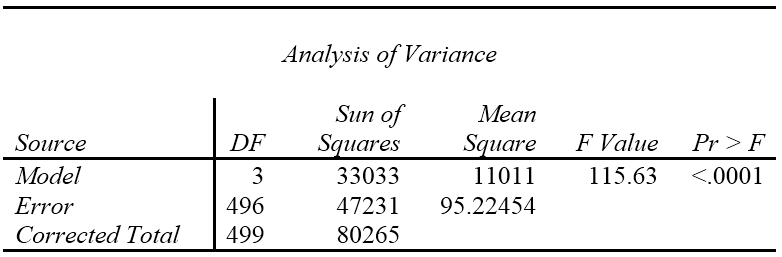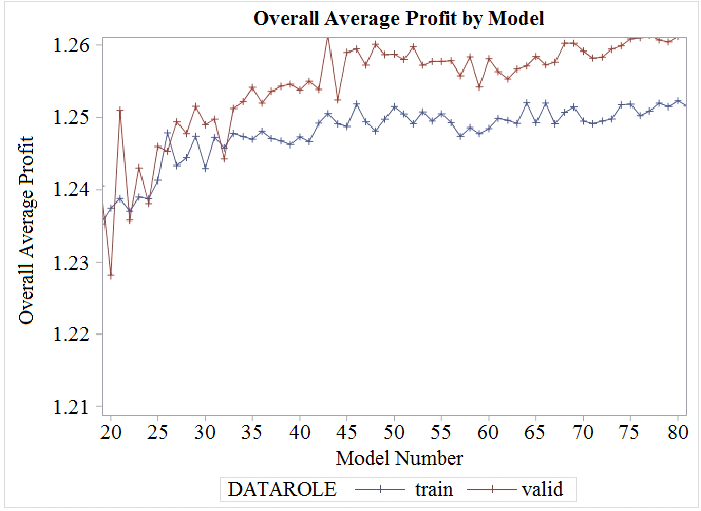# SAS A00-240 SAS Statistical Business Analysis SAS9: Regression and Model Exam Practice Test

Page:    1 / 14
Total 99 questions

### Question 1

The selection criterion used in the forward selection method in the GLMSELECT procedure is:

### Question 2

Refer to the REG procedure output:Calculate the coefficient of determination, R-Square.

Enter your numeric answer in the space below. Round to 4 decimal places (example: n.nnnn).

See Below Explanation:

### Question 3

When working with smaller data sets (N<200), which method is preferred to perform honest assessment?

### Question 4

A researcher is planning a logistic regression to model the probability of disease occurrence. The researcher determines the rate of disease occurrence in the population is 1%.

For which of the following would this study be a candidate?

### Question 5

The PROC LOGISTIC options SELECTION=SCORE and BEST=2 are used in a MODEL statement to generate a series of predictive models. The models are assigned numbers in order from 1 to 99 reflecting the fact that there are 50 candidate input variables. Results from the collection of derived models are used to generate the following plot of overall average profit by model number. Results are restricted to models with at least 9 inputs and at most 40 inputs.The maximum value for the training data occurs for model number 46, and the maximum value for the validation data occurs for model number 43.

If you base model selection solely on overall average profit, what is the correct choice?